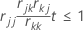# Methods in Fit Poisson Model

Select the method or formula of your choice.

## Factor/covariate pattern

Describes a single set of factor/covariate values in a data set. Minitab calculates event probabilities, residuals, and other diagnostic measures for each factor/covariate pattern.

For example, if a data set includes the factors gender and race and the covariate age, the combination of these predictors may contain as many different covariate patterns as subjects. If a data set only includes the factors race and sex, each coded at two levels, there are only four possible factor/covariate patterns. If you enter your data as frequencies, or as successes, trials, or failures, each row contains one factor/covariate pattern.

## How Minitab removes highly correlated predictors from the regression equation in Fit Poisson Model

Let rij be the element in the current swept matrix associated with Xi and Xj.

Variables are entered or removed one at a time. Xk is eligible for entry if it is an independent variable not currently in the model with rkk ≥ 1 (tolerance with a default of 0.0001) and also for each variable Xj that is currently in the model,To remove highly correlated predictors from a regression equation, Minitab does the following steps:
1. Minitab performs the SWEEP method on the correlation matrix, R, treating X1 … Xp as if they are random variables.
2. For any continuous predictor, Minitab compares the element rkk with the tolerance; rkk ≥ tolerance, where k = 1 to p.
3. For each variable Xj currently in the model, Minitab checks that (rjj – rjk * (rkj / rkk)) * tolerance ≤ 1.
###### Note

Where rkk, rjk, rjj are the corresponding diagonal and off diagonal elements for Xj and Xk variables after k step SWEEP operations.

4. Otherwise, the predictor fails the test and is removed from the model.
###### Note

The default tolerance value is 8.8e–12.

###### Note

You can use the TOLERANCE subcommand with the GZLM session command to force Minitab to keep a predictor in the model that is highly correlated with a different predictor. However, lowering the tolerance can be dangerous, possibly producing numerically inaccurate results.

By using this site you agree to the use of cookies for analytics and personalized content.  Read our policy